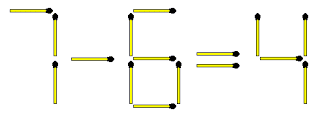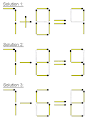Drop down Tab menu for Site

FIGURE OUT PUZZLES - matchstick puzzles (02)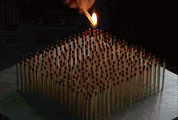QUESTION 1
Ōe is a town in Yamagata, Japan.
Remove 2 to leave 1.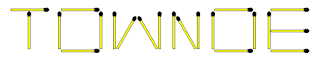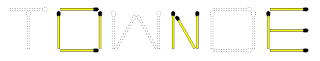QUESTION 2
MOVE 3 matchsticks to make the equation true. Can you find all 3 solutions?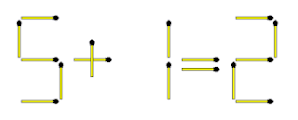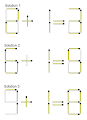QUESTION 3
TAKE AWAY 3 matchsticks to form the SMALLEST possible number?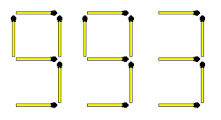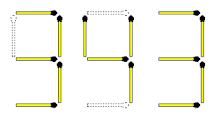QUESTION 4
The vertical matchsticks on the scale all have the same weight. To balance the scale you need to add 1 more matchstick. Where would you place it?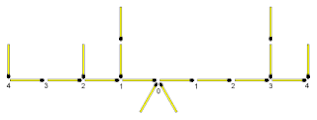In the original puzzle:
Left of balancing point: 4 + 2 + 1 + 1 = 8 units
Right of balancing point: 3 + 3 + 4 = 10 units
From the above we can see we need to add 2 more units to the left of the balancing point. This is done by placing the matchstick as shown in the solution.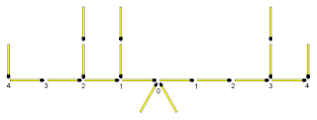QUESTION 5
Replace the "?" with the next number in the sequence.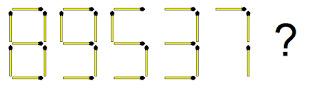Each figure has one node (connection of 2 or 3 matchsticks) less than the previous one.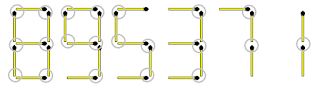QUESTION 6
The equation above is not true. Move only 1 matchstick to make it true.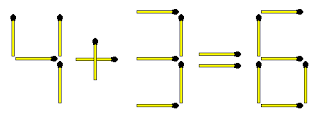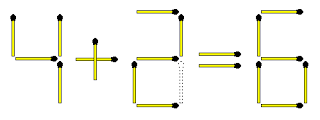QUESTION 7
Move only 1 matchstick to make the horse standing on its hind legs.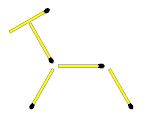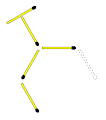QUESTION 8
Move 3 matchsticks to make the equation true.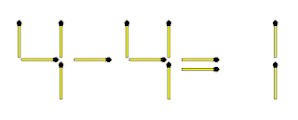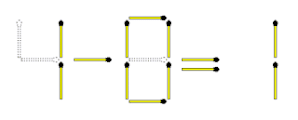QUESTION 9
Everything did not go well for this out of control skier - it ended with his head in the snow and skis in the air!
Move only 3 matchsticks to show how his skiing ended.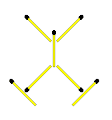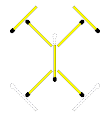QUESTION 10
Take away 3 matchsticks to make the above equation true. Can you find both solutions?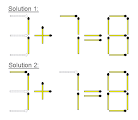QUESTION 11
What is the next figure in the sequence above?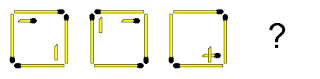1. The 4 matchsticks on the outside move clockwise (or anti-clockwise) one corner at a time.
2. The broken matchstick with head moves clockwise one corner at a time retaining the horizontal position with matchstick-head pointing to the right.
3. The broken matchstick without head moves back and forth from the bottom right corner to the top left retaining the vertical position.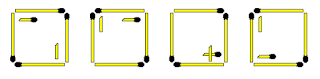QUESTION 12
Move only 1 matchstick to make the equation true. Can you find both solutions?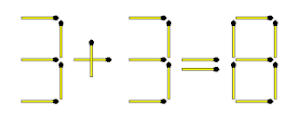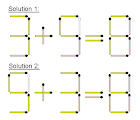QUESTION 13
Take away 3 matchsticks to make the above equation true. There are 3 correct solutions. Can you find them all?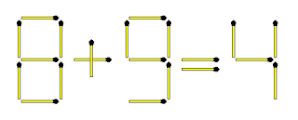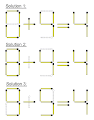QUESTION 14
Move 4 matchsticks to make the equation true.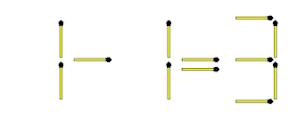QUESTION 15
Move 2 matchsticks to create 2 squares. The 2 squares must be different in size.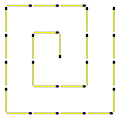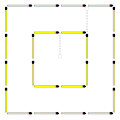QUESTION 16
This puzzle is similar to but different from Number 15.
Move 3 matchsticks to create 3 squares. The 3 squares must be different in size.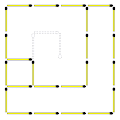QUESTION 17
Take away 5 matchsticks to make the above equation true.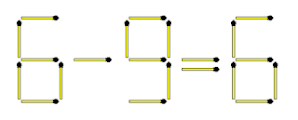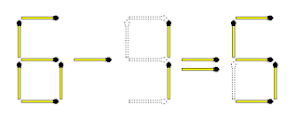QUESTION 18
The equation above is not true. Take away 2 matchsticks to make it true.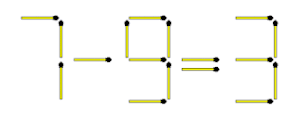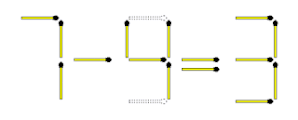QUESTION 19
The above layout shows a distance of 100 metres. Move 2 matchsticks to convert it to the smallest possible distance.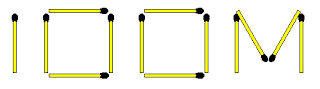10 millimetres. There are 1000 millimetres in 1 metre.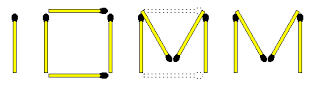QUESTION 20
Move 3 matchsticks to make the equation true.